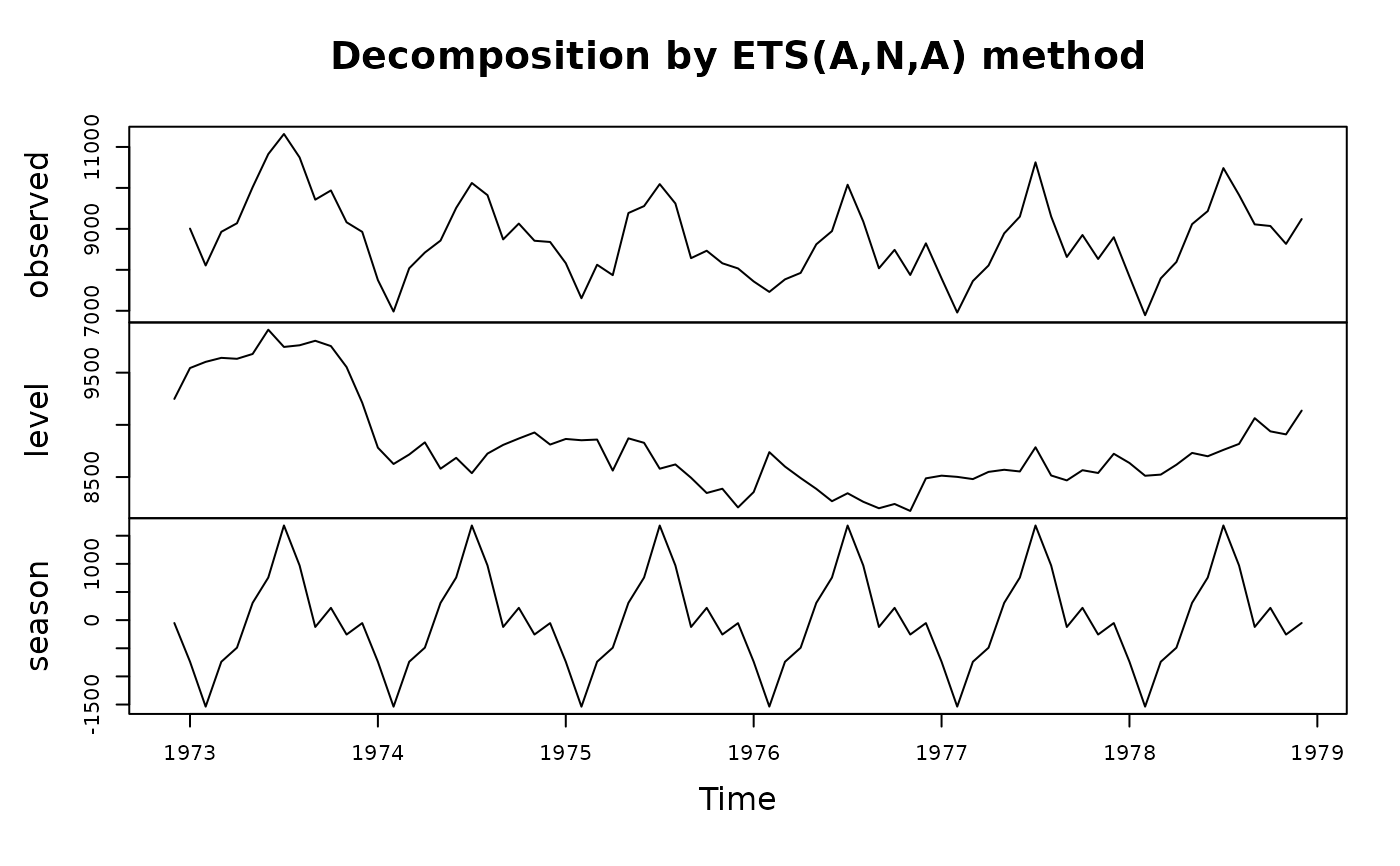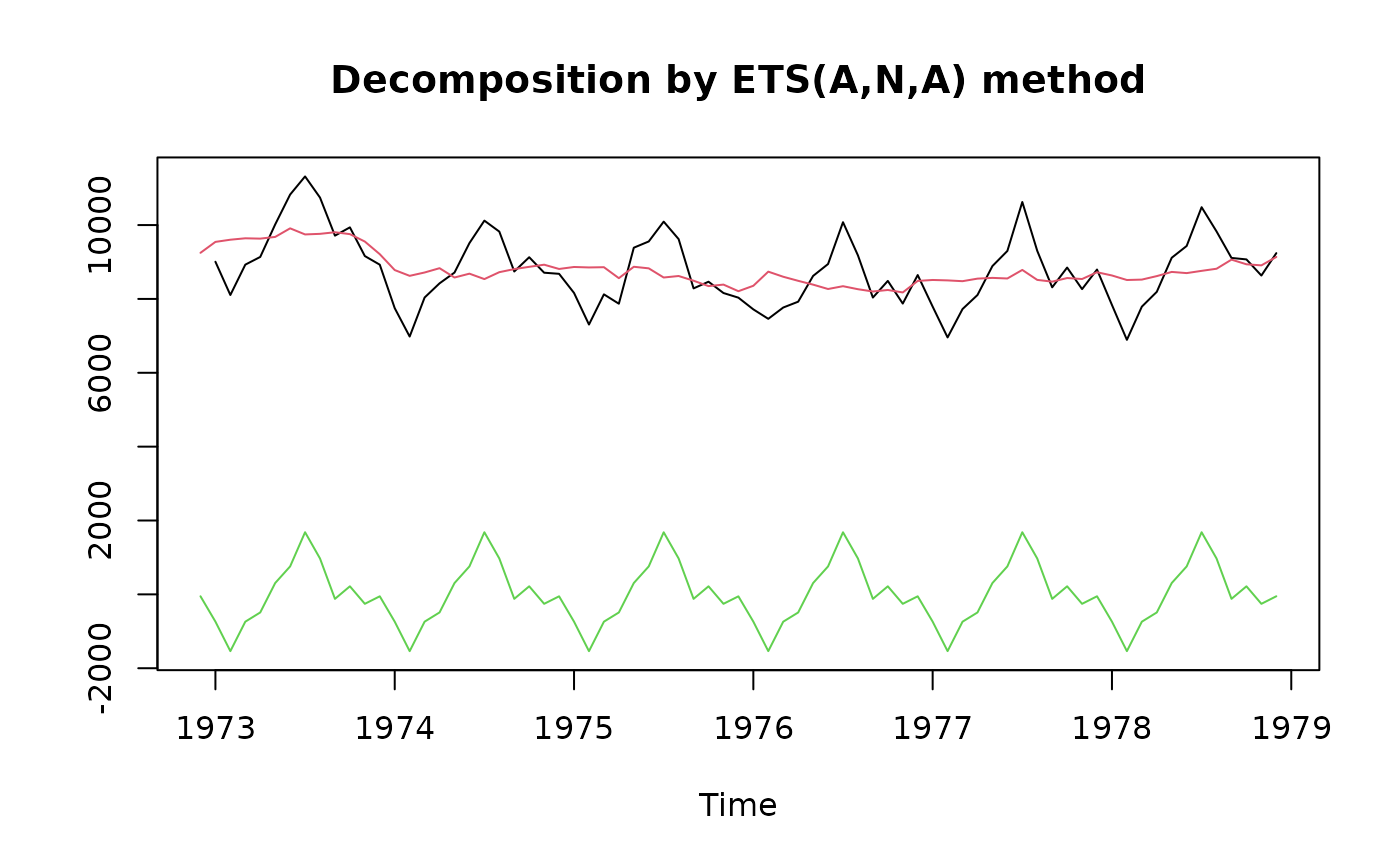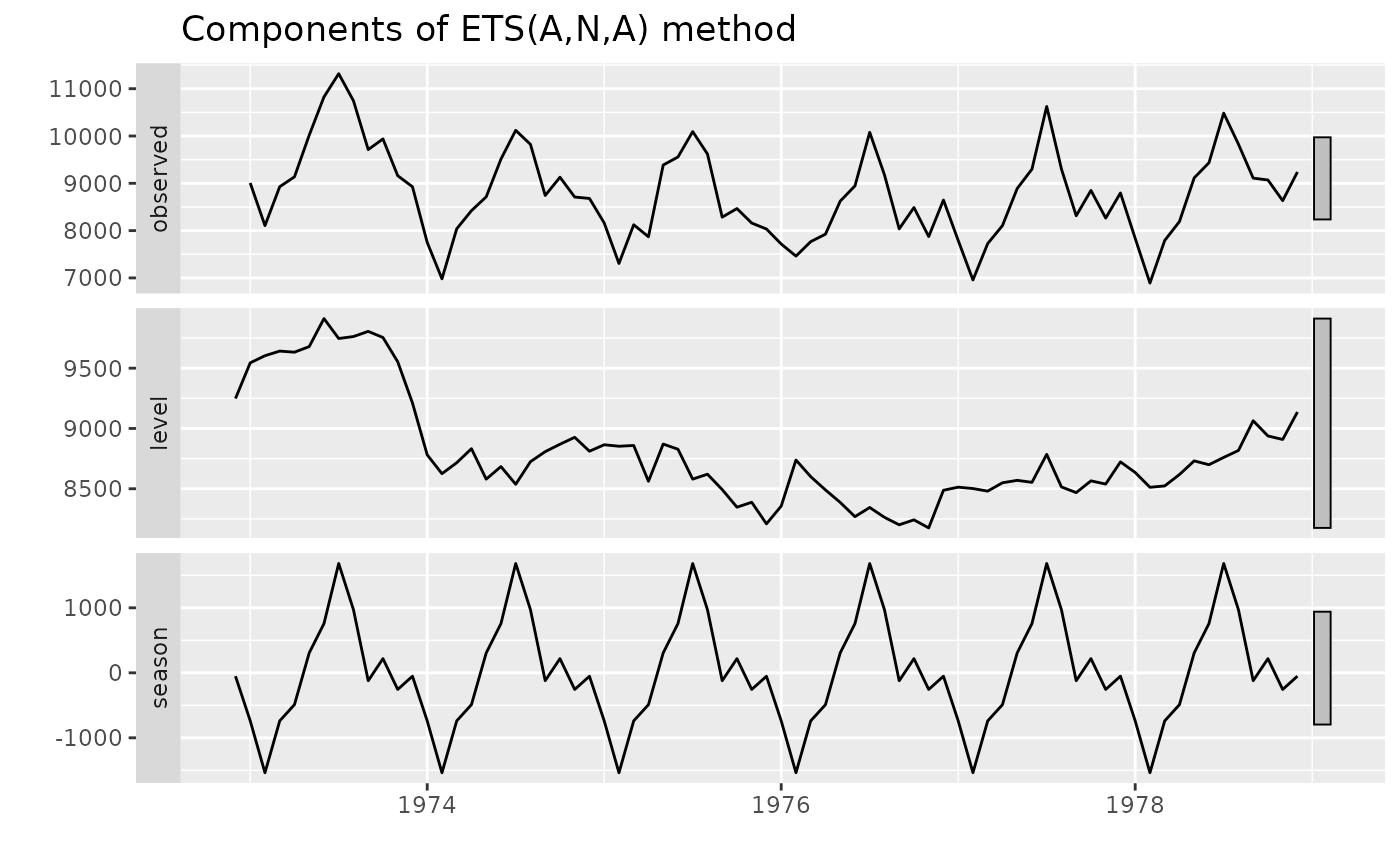Produces a plot of the level, slope and seasonal components from an ETS model.

# S3 method for ets
plot(x, ...)

# S3 method for ets
autoplot(object, range.bars = NULL, ...)

## Arguments

x Object of class “ets”. Other plotting parameters to affect the plot. Object of class “ets”. Used for ggplot graphics (S3 method consistency). Logical indicating if each plot should have a bar at its right side representing relative size. If NULL, automatic selection takes place.

## Value

None. Function produces a plot

## Details

autoplot will produce an equivalent plot as a ggplot object.

ets

## Author

Rob J Hyndman & Mitchell O'Hara-Wild

## Examples


fit <- ets(USAccDeaths)
plot(fit)plot(fit,plot.type="single",ylab="",col=1:3)library(ggplot2)
autoplot(fit)